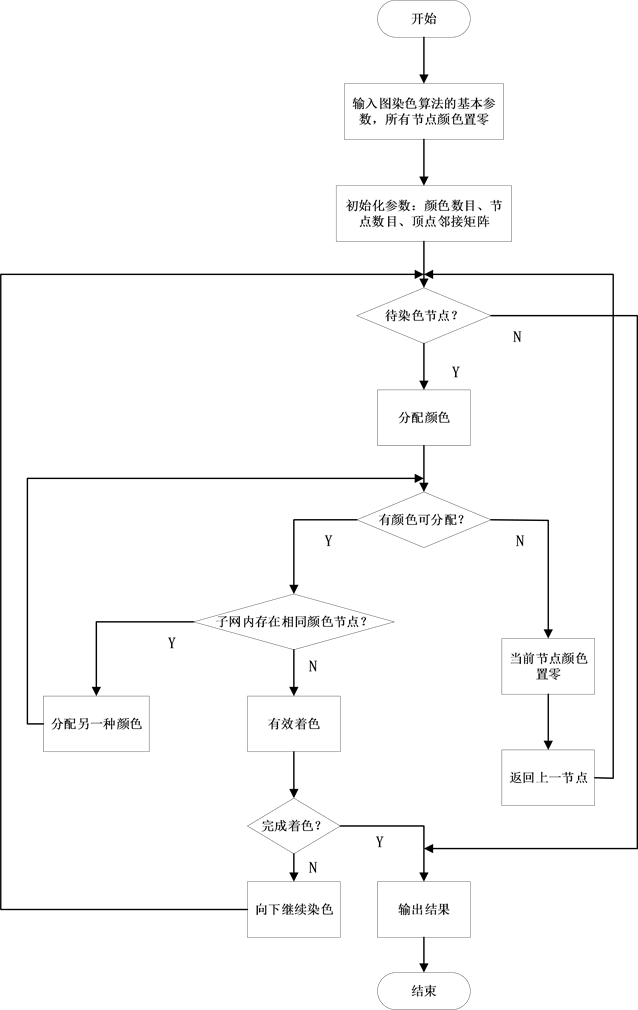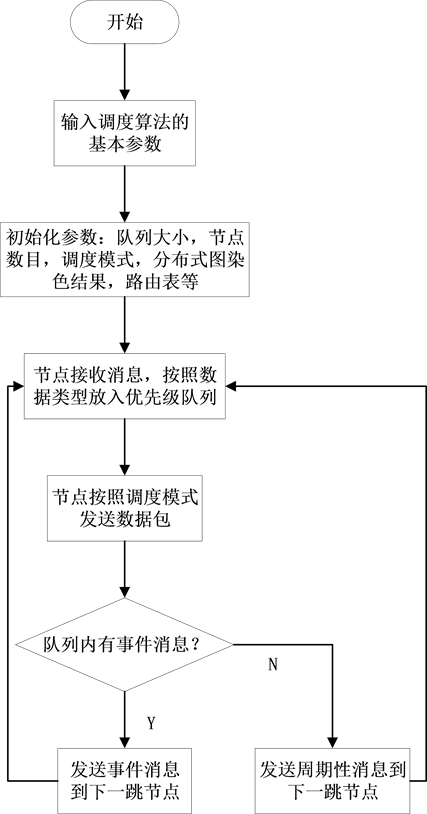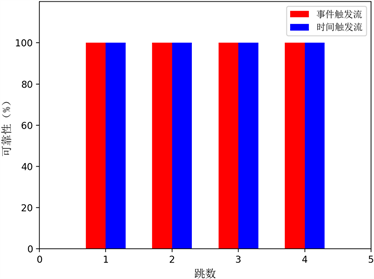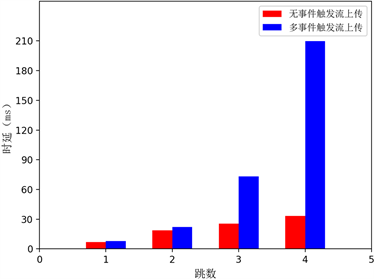# 工业无线网络TSN实时优先队列调度算法研究Research on TSN Real-Time Priority Queue Scheduling Algorithm for Industrial Wireless Networks

• 全文下载: PDF(614KB)    PP.326-333   DOI: 10.12677/SEA.2019.86040
• 下载量: 222  浏览量: 360

With the development of industrial and information technology, industrial wireless networks as the basic technology of industrial Internet of Things, have received more and more attention from the industry. It replaces the wired connection with wireless communication, which greatly reduces the cost of installation and maintenance. In complex industrial environment, it provides a flexible configuration solution for field devices, which provides a powerful technical impetus for the development of Industry 4.0. Based on the asynchronous scheduling mode, the distributed graph coloring algorithm and the priority queue scheduling algorithm are used to realize the asynchro-nous scheduling of multi-hop networks. The validity of the scheduling algorithm is verified on the contiki platform. The experimental result shows that the algorithm guarantees real-time commu-nication of event-triggered flows and time-triggered flows.

1. 引言

2. 系统模型Figure 1. Industrial wireless network topologyTable 1. Each symbol represents meaning

3. 问题陈述

$\mathrm{min}\underset{i=1}{\overset{N}{\sum }}\left(ti-si\right)$ (1)

$\underset{i=1}{\overset{N}{\sum }}{f}_{i,j}=1\text{\hspace{0.17em}}\text{\hspace{0.17em}}\text{\hspace{0.17em}}\underset{i=1}{\overset{N}{\sum }}{f}_{j,i}=0$ (2)

$\underset{i=1}{\overset{V}{\sum }}{f}_{i,\mathrm{sin}k}=1\text{\hspace{0.17em}}\text{\hspace{0.17em}}\text{\hspace{0.17em}}\underset{i=1}{\overset{V}{\sum }}{f}_{\mathrm{sin}k,i}=0$ (3)

$m\le M$ (4)

$R\ge Nv$ (5)

$C\ge N$ (6)

$\omega \ge \tau TN$ (7)

4. 基于优先级队列的免同步调度算法

4.1. 分布式图染色

4.2. 优先级队列调度Figure 2. Flow chart of distributed graph coloring algorithmFigure 3. Flow chart of priority queue scheduling algorithm

5. 结果评估Figure 4. Reliability experiment resultsFigure 5. Real-time experimental results

6. 结论

  Saifullah, A., Xu, Y., Lu, C., et al. (2010) Real-Time Scheduling for Wireless HART Networks. In: 31st IEEE Real-Time Systems Symposium, IEEE, Piscataway, 150-159. https://doi.org/10.1109/RTSS.2010.41  Quang, P.T.A. and Kim, D.S. (2013) Throughput-Aware Routing for Industrial Sensor Networks: Application to ISA100.11a. IEEE Transactions on Industrial Informatics, 10, 351-363. https://doi.org/10.1109/TII.2013.2255617  Zhang, X., Liang, W., Zheng, M., et al. (2009) Distributed and Dynamic TDMA Channel Scheduling Algorithm for WIA-PA. IEEE International Conference on Intelligent Computing and Intelligent Systems, Vol. 3, 462-466.  Chen, Y., Zhang, H., Fisher, N., et al. (2018) Probabilistic Per-Packet Real-Time Guarantees for Wireless Networked Sensing and Control. IEEE Transactions on Industrial Informatics, 14, 2133-2145. https://doi.org/10.1109/TII.2018.2795567  Ma, Y., Guo, J., Wang, Y., et al. (2019) Optimal Dynamic Scheduling of Wireless Networked Control Systems. In: Proceedings of the 10th ACM/IEEE International Conference on Cyber-Physical Systems, ACM, New York, 77-86. https://doi.org/10.1145/3302509.3311040  Kotsiou, V., Papadopoulos, G.Z., Zorbas, D., et al. (2019) Blacklist-ing-Based Channel Hopping Approaches in Low-Power and Lossy Networks. IEEE Communications Magazine, 57, 48-53. https://doi.org/10.1109/MCOM.2018.1800362  Wang, Z., Qin, X., Liu, B., et al. (2019) Joint Data Sampling and Link Scheduling for Age Minimization in Multihop Cyber-Physical Systems. IEEE Wireless Communications Letters, 8, 765-768. https://doi.org/10.1109/LWC.2019.2891598